### Anna Grossnickle HinesHomeGuide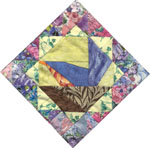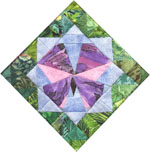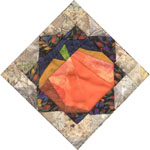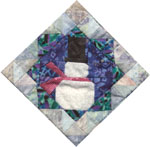Quilts in the Classroom

Let's Do Math to PIECES!
using the book, PIECES: A YEAR IN POEMS AND QUILTS

Designing With Half-square Triangles

Let's play with lines!

• Take a square. Draw a line to divide it half diagonally.
• What happens?
• Quilters call these triangles half-square triangles.  They use them a lot.
• In PIECES three quilts use half-square triangles.
• Can you find them?
• Two use just a few half-square triangles, but one uses lots of them.
• It was fun to design with half-square triangles.
• There are so many possibilities! Want to try a few?
• Take two squares. Draw the lines to divide them in half diagonally.
• How many different ways can you put them together?
• Take four squares and divide them in half diagonally.
•  Now how many ways can you put them together?
• Try this with 16 squares.
• How many different designs can you make?
• Try 25 squares.
• What happens?  Do you run into any difficulties or limitations?
• Why?
• Try 36 squares.
• Now what happens?
• Isn't that interesting?
• Do you know about symmetry?
• When something is symmetrical, you can put a line through the center and both halves will be just the same.
• Actually the two halves will be exact opposites, or mirror images of one another.
• Can you think of anything else that is symmetrical?
• Nature uses lots of symmetry. Keep an eye open for examples.
• Can you make a symmetrical design with 8 half square triangle blocks?
• Can you make a square design?
• Can you make a symmetrical design with 9 half-square triangle blocks?
• Can you make a square design?
• What's the next number of half-square triangle blocks you need to make a symmetrical design?
• What's the next number you need to make a square design?
• Are designs using half-square triangles always symmetrical?
• Are symmetrical designs always square?
• What does it take to make a design that is both square and symmetrical?
• What happens if you use plain squares instead of triangle-squares?
• When a design is not symmetrical we call it asymmetrical.
• Can you make an asymmetrical design with 9 half-square triangle blocks?
• Can you do it with 4?  ....16?  ....36?
Want to try some more symmetrical and asymmetrical designs?  Let's add color!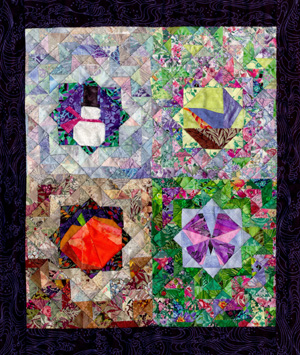For my Pieces Quilt I turned the grid so sides of the squares are at an angle and the diagonals of the squares are vertical (up and down) or horizontal (across). Quilters say the squares are on point, because they sit on their points instead of their flat bottoms.   This makes counting them a little complicated, especially around the edges where parts of them are sewn into the borders, but here's the design I used. For this quilt I started with a grid that has the squares at an angle.  Quilters say these squares are "on point" because they sit on their points instead of their flat bottoms.       To print this grid click on the image.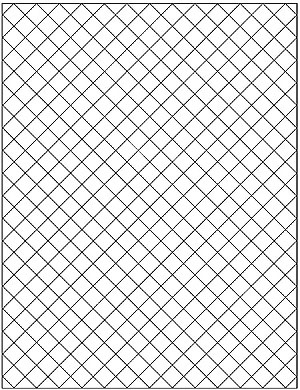When I first designed my quilt I was using this grid. Do you see how the triangles go?  In the first row across they are all vertical, right?  And in the second row they are all...which way? And in the third row? And fourth? I drew my grid and started coloring it in on my computer and didn't even realize I could change the direction of the triangles.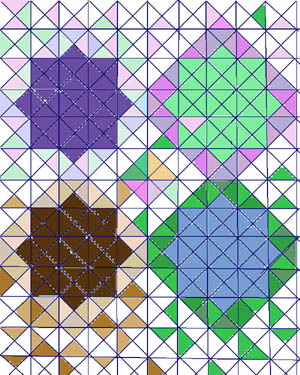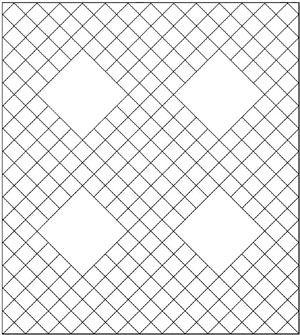Here is a grid with the squares still whole. On this grid I drew a horizontal or vertical line through each square to show how I wanted to sew my half-square triangles. How many rows are there? How many columns? It's tricky to count because they are staggered, with a half-square on the end of every other row and every other column. Each of these half-squares is a triangle, right?  And if you put the two on the ends of each row together you get what?  One whole square for the row, right? But what happens on the columns? Can you figure out how many squares I needed to make?  How many triangles? The big squares are where I put the seasonal symbols.  If I were to put squares in there, how many would it take for each one?  How many for all four? This is the way I chose to put my triangles. Even with that much decided there are still lots of possibilities.  What will happen when I start adding color?   To print either of these grids to make your own   design,  click on the image. To print my Pieces Quilt design with the symbols in place click here.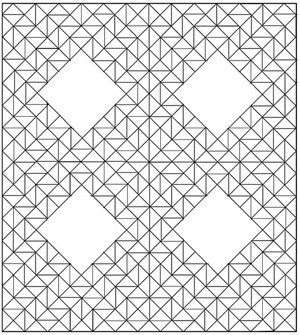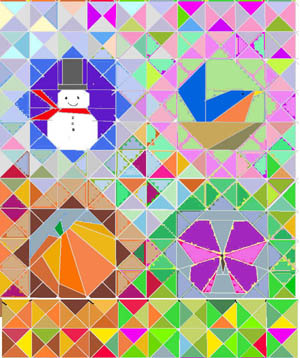Here is my computer design for this quilt.  It was the first time I tried designing on the computer and as you can see I wasn't very good.  I'm still not REALLY good, but I'm getting better.  I'm designing a lot of the quilts for my next book on the computer.  It is a good way to experiment with color and check out a lot of possibilities  before I cut the fabric. Do you want to design a quilt using half-square triangles in a program such as Corel Draw, Paintshop Pro,  PhotoShop or others that let you "fill" spaces with colors? Download this jpeg grid file, open it in your software program and have fun! More about how I made this quilt. Seasonal symbol designs